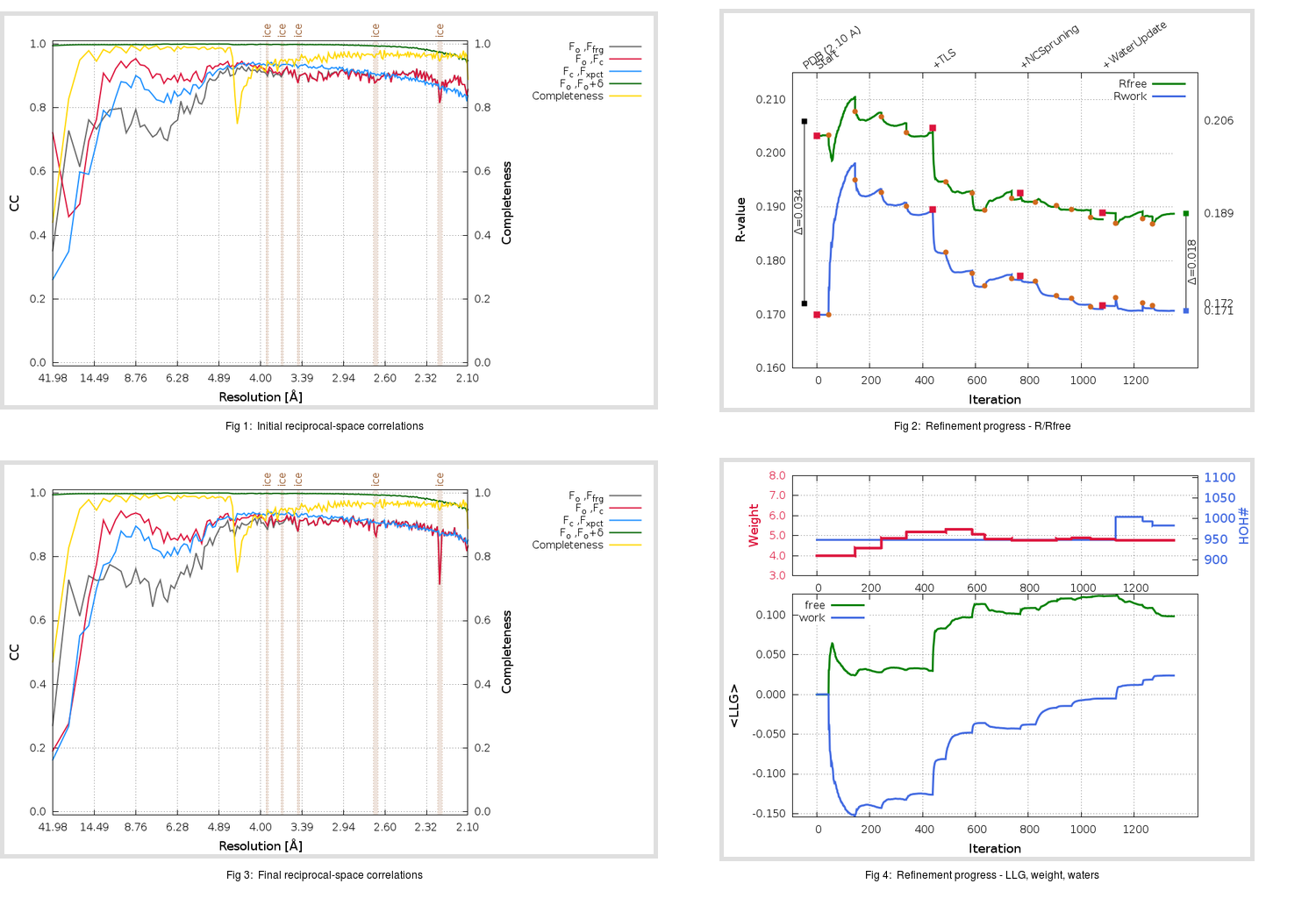Content:

```    Diffraction limits & principal axes of ellipsoid fitted to diffraction cut-off surface:
2.072         0.7183  -0.3397   0.6071       0.721 a* - 0.512 b* + 0.467 c*
2.060         0.3318   0.9343   0.1303       0.294 a* + 0.850 b* - 0.437 c*
2.081        -0.6115   0.1078   0.7838      -0.540 a* + 0.208 b* + 0.816 c*
```

## Deposited

` `
 Date deposited Date data collection Resolution R, Rfree 20100922 20100501 2.10 0.1710 0.2060

Molprobity (CCP4 7.0 version) summary:

```Ramachandran outliers =   0.08 %
favored =  97.16 %
Rotamer outliers      =   4.00 %
C-beta deviations     =     0
Clashscore            =   8.28
RMS(bonds)            =   0.0075
RMS(angles)           =   1.05
MolProbity score      =   2.06
Resolution            =   2.10
R-work                =   0.1710
R-free                =   0.2060
```

```Number of waters      =   948

<B> (all atoms) =   35.96 ( sd =   16.26 ) for      10982 non-hydrogen atoms
<B>   (protein) =   35.61 ( sd =   16.71 ) for       9986 non-hydrogen atoms
<B>     (water) =   39.13 ( sd =   10.02 ) for        948 non-hydrogen atoms
<B>    (others) =   44.23 ( sd =   12.44 ) for         48 non-hydrogen atoms

B min/max       (all non-hydrogen atoms) =    9.49 /  130.82
B min/max   (protein non-hydrogen atoms) =    9.57 /  130.82
B min/max     (water non-hydrogen atoms) =    9.49 /   67.77
B min/max     (other non-hydrogen atoms) =   17.95 /   66.21
```

## BUSTER (re-)refinement

` `

Molprobity (CCP4 7.0 version) summary:

```Ramachandran outliers =   0.00 %
favored =  98.38 %
Rotamer outliers      =   4.47 %
C-beta deviations     =     0
Clashscore            =   3.64
RMS(bonds)            =   0.0120
RMS(angles)           =   1.52
MolProbity score      =   1.65
Resolution            =   2.10
R-work                =   0.1707
R-free                =   0.1888
```

```Number of waters      =   983

<B> (all atoms) =   38.94 ( sd =   17.05 ) for      11017 non-hydrogen atoms
<B>   (protein) =   38.15 ( sd =   17.07 ) for       9986 non-hydrogen atoms
<B>     (water) =   45.95 ( sd =   14.28 ) for        983 non-hydrogen atoms
<B>    (others) =   58.39 ( sd =   17.38 ) for         48 non-hydrogen atoms

B min/max       (all non-hydrogen atoms) =   10.70 /  118.36
B min/max   (protein non-hydrogen atoms) =   10.70 /  113.58
B min/max     (water non-hydrogen atoms) =   11.11 /  118.36
B min/max     (other non-hydrogen atoms) =   23.39 /   75.10
```

Refinement progression:Results:

` `
 File Remark 3OXU_aB_refine.01_04_refine.pdb.gz exact refinement commands are in header 3OXU_aB_refine.01_04_refine.mtz.gz including original deposited data and several re-refinement map coefficients 3OXU_aB_refine.01_04_BUSTER_model.cif.gz including any non-standard compound restraints 3OXU_aB_refine.01_04_BUSTER_refln.cif.gz